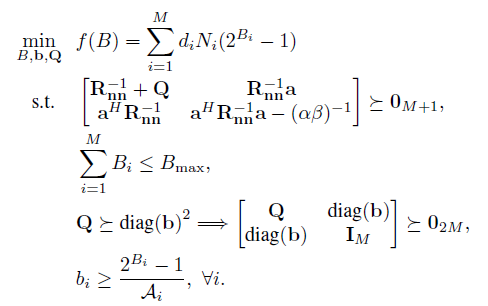# CVX Index exceeds matrix dimensions?

Hello, everyone. I have an optimization problem as followsThen, I wrote the codes in MATLAB, as follows

Why matlab always shows the error like thisI don’t understand the reason, because I have checked that there is not problem with matrix dimension.
So who can give me advice? thanks very much.

2 posts were merged into an existing topic: CVX_SDP Index exceeds matrix dimensions problem